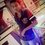# PROVE IT !!!if m times the m term of an A.P. is equal to the n times the n term and (m) is not equal to (n) show that (m+n) term is 0 .Note by Palash Som
6 years, 10 months ago

This discussion board is a place to discuss our Daily Challenges and the math and science related to those challenges. Explanations are more than just a solution — they should explain the steps and thinking strategies that you used to obtain the solution. Comments should further the discussion of math and science.

When posting on Brilliant:

• Use the emojis to react to an explanation, whether you're congratulating a job well done , or just really confused .
• Ask specific questions about the challenge or the steps in somebody's explanation. Well-posed questions can add a lot to the discussion, but posting "I don't understand!" doesn't help anyone.
• Try to contribute something new to the discussion, whether it is an extension, generalization or other idea related to the challenge.

MarkdownAppears as
*italics* or _italics_ italics
**bold** or __bold__ bold
- bulleted- list
• bulleted
• list
1. numbered2. list
1. numbered
2. list
Note: you must add a full line of space before and after lists for them to show up correctly
paragraph 1paragraph 2

paragraph 1

paragraph 2

[example link](https://brilliant.org)example link
> This is a quote
This is a quote
    # I indented these lines
# 4 spaces, and now they show
# up as a code block.

print "hello world"
# I indented these lines
# 4 spaces, and now they show
# up as a code block.

print "hello world"
MathAppears as
Remember to wrap math in $$ ... $$ or $ ... $ to ensure proper formatting.
2 \times 3 $2 \times 3$
2^{34} $2^{34}$
a_{i-1} $a_{i-1}$
\frac{2}{3} $\frac{2}{3}$
\sqrt{2} $\sqrt{2}$
\sum_{i=1}^3 $\sum_{i=1}^3$
\sin \theta $\sin \theta$
\boxed{123} $\boxed{123}$

Sort by:

Let first term of A.P. be a and common difference be d. Hence we can write, $m[ a + (m-1)d ] = n[ a + (n-1)d ]$ $\therefore am + md(m-1) = an + nd(n-1)$ $\therefore am-an +md(m-1) - nd(n-1) =0$ $\therefore a(m-n) + d( m(m-1) - n(n-1) ) = 0$ $\therefore a(m-n) + d ( m^2 - n^2 -m + n ) = 0$ $\therefore a(m-n) + d[ (m-n)(m+n) - (m-n) ] =0 \cdots (i)$ As we know that $m \neq n$ , so we can write that $m-n \neq 0$ Hence, we can divide the above equation $(i)$ by $m-n$ then we get, $a + [ (m+n) - 1 ]d = 0 \cdots (ii)$ Now the $(m+n)^{th}$ of the A.P. is equal to $a + [(m+n) -1 ]d \cdots (iii)$ From $(ii) and (iii)$ we get ,$a + [(m+n) -1] d=0$ i.e. $(m+n)^{th}$ term of A.P. is $0$ Hence, proved.

- 6 years, 10 months ago

- 6 years, 10 months ago

nicely done thnx..

- 6 years, 10 months ago

Let $x_a$ represent the $a^{th}$ term of the progression. Since this is an arithmetic progression, there exist $c,d \in \mathbb{R}$ such that $x_a = c*a + d$ for all $a \in \mathbb{N}$. Then, $m*x_m = n*x_n$ $m(cm+d) = n(cn+d)$ $cm^2+dm = cn^2 + dn$ $c(m^2-n^2)+d(m-n)=0$

Since $m \neq n$, we can divide by $m - n$:

$c(m+n) + d = 0$

Therefore, the $(m+n)^{th}$ term is 0.

- 6 years, 10 months ago

@ariel gershon what do c denotes here cos in india we have a different way to represent any term i.e. a+(n-1)*d where a is the first term n is the no. of term to find and d is the difference

- 6 years, 10 months ago

$c$ and $d$ are just constants. I agree, your formula is more commonly used, but you can rewrite it as $d*n + (a-d)$, which fits the formula I used. I realize now that my method isn't that different from Kunal's; but I posted my solution before seeing his.

- 6 years, 10 months ago

thnx a lot .. :-)

- 6 years, 10 months ago

Bro dis sum is of 10th ssc hot sums ._. Don't copy :/

- 6 years, 9 months ago

hey @vedant this one is a question in a book RS AGGARWAL

- 6 years, 8 months ago